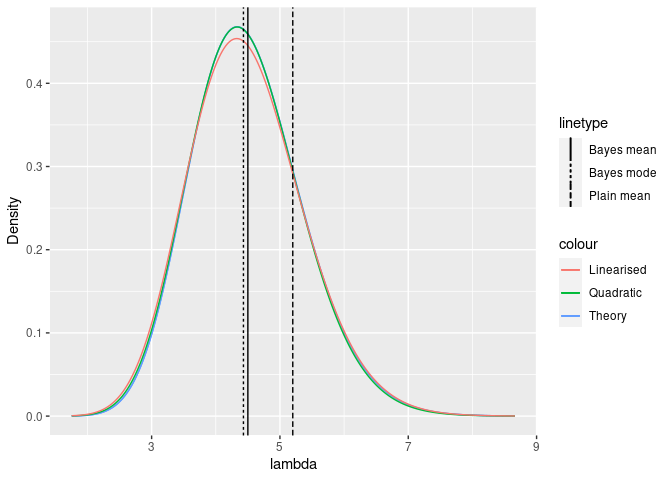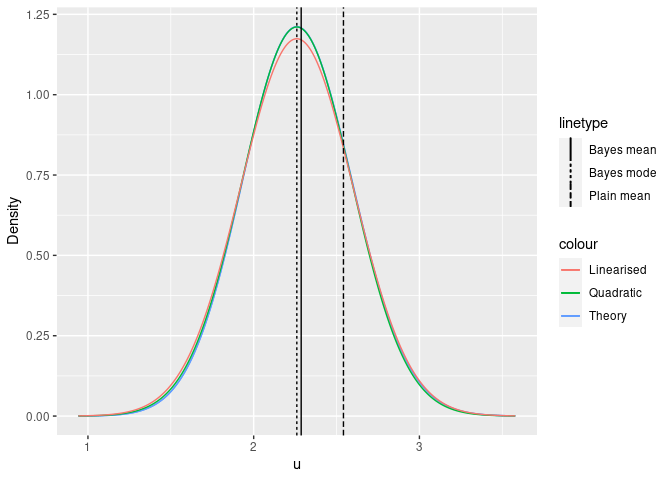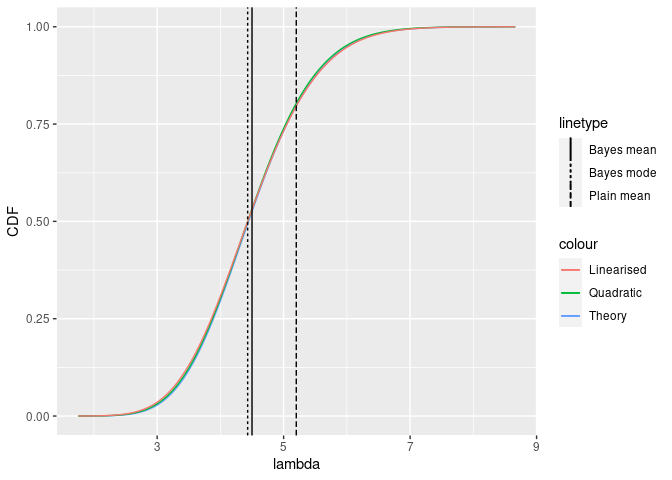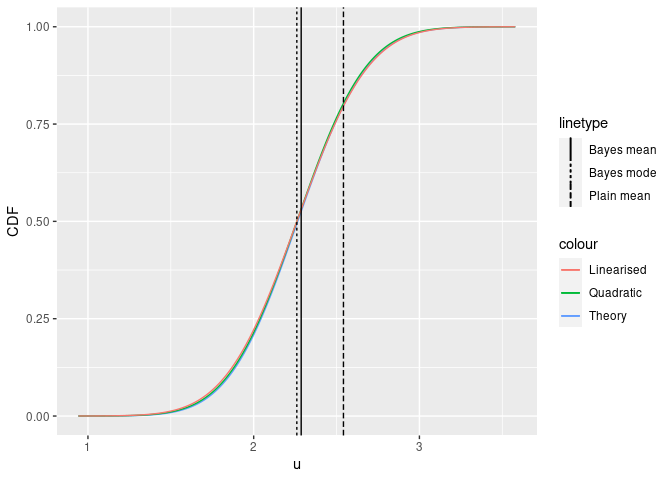# Nonlinear model approximation

See the method vignette for details of the general inlabru method. Here, we’ll take a look at a small toy example to see the difference between a few posterior approximation methods.

Note: This vignette is not completed yet.

## A small toy problem

Hierarchical model: \begin{aligned} \lambda &\sim \mathsf{Exp}(\gamma) \\ (y_i|\lambda) &\sim \mathsf{Po}(\lambda), \text{ independent across i=1,\dots,n} \end{aligned} With $$\overline{y}=\frac{1}{n}\sum_{i=1}^n y_i$$, the posterior density is \begin{aligned} p(\lambda | \{y_i\}) &\propto p(\lambda, y_1,\dots,y_n) \\ &\propto \exp(-\gamma\lambda) \exp(-n\lambda) \lambda^{n\overline{y}} \\ &= \exp\{-(\gamma+n)\lambda\} \lambda^{n\overline{y}}, \end{aligned} which is the density of a $$\mathsf{Ga}(\alpha = 1+n\overline{y}, \beta = \gamma+n)$$ distribution.

## Latent Gaussian predictor version

Introducing a latent Gaussian variable $$u\sim\mathsf{N}(0,1)$$, the model can be reformulated as \begin{aligned} \lambda(u) &=-\ln\{1-\Phi(u)\}/\gamma \\ (y_i|u) &\sim \mathsf{Po}(\lambda(u)) \end{aligned} We will need some derivatives of $$\lambda$$ with respect to $$u$$: \begin{aligned} \frac{\partial\lambda(u)}{\partial u} &= \frac{1}{\gamma}\frac{\phi(u)}{1-\Phi(u)} = \lambda'(u) \\ \frac{\partial^2\lambda(u)}{\partial u^2} &= - \frac{1}{\gamma}\frac{\phi(u)}{1-\Phi(u)} \left( u + \frac{\phi(u)}{1-\Phi(u)} \right) = -\lambda'(u)\left\{u-\gamma\lambda'(u)\right\} \\ \frac{\partial\ln\lambda(u)}{\partial u} &= \frac{1}{\lambda(u)} \frac{\partial\lambda(u)}{\partial u} =\frac{1}{-\ln\{1-\Phi(u)\}} \frac{\phi(u)}{1-\Phi(u)} = \frac{\lambda'(u)}{\lambda(u)} \\ \frac{\partial^2\ln\lambda(u)}{\partial u^2} &= \frac{1}{\lambda(u)} \frac{\partial^2\lambda(u)}{\partial u^2} -\frac{1}{\lambda(u)^2} \left(\frac{\partial\lambda(u)}{\partial u}\right)^2 = \frac{-\lambda'(u)\{u - \gamma\lambda'(u)\}}{\lambda(u)} - \frac{\lambda'(u)^2}{\lambda(u)^2}\\ &= -\frac{\lambda'(u)}{\lambda(u)}\left\{ u - \gamma\lambda'(u) +\frac{\lambda'(u)}{\lambda(u)} \right\} \end{aligned}

lambda <- function(u, gamma) {
-pnorm(u, lower.tail = FALSE, log.p = TRUE) / gamma
}
lambda_inv <- function(lambda, gamma) {
qnorm(-lambda * gamma, lower.tail = FALSE, log.p = TRUE)
}
D1lambda <- function(u, gamma) {
exp(dnorm(u, log = TRUE) - pnorm(u, lower.tail = FALSE, log.p = TRUE)) / gamma
}
D2lambda <- function(u, gamma) {
D1L <- D1lambda(u, gamma)
-D1L * (u - gamma * D1L)
}
D1log_lambda <- function(u, gamma) {
D1lambda(u, gamma) / lambda(u, gamma)
}
D2log_lambda <- function(u, gamma) {
D1logL <- D1log_lambda(u, gamma)
-D1logL * (u - gamma * D1lambda(u, gamma = gamma) + D1logL)
}

## Latent Gaussian posterior approximations

A basic approximation of the posterior distribution for $$\lambda$$ given $$y$$ can be defined as a deterministic transformation of a Gaussian distribution obtained from a 2nd order Taylor approximation of $$\ln p(u|\{y_i\})$$ at the posterior mode $$u_0$$ of $$p(u|\{y_i\})$$. The needed derivatives are \begin{aligned} \frac{\partial\ln p(u|\{y_i\})}{\partial u} &= \frac{\partial\ln\phi(u)}{\partial u} - n\lambda'(u) + n\overline{y}\frac{\lambda'(u)}{\lambda(u)} = -u + n\frac{\lambda'(u)}{\lambda(u)}\left\{ \overline{y} - \lambda(u) \right\} \\ \frac{\partial^2\ln p(u|\{y_i\})}{\partial u^2} &= -1 - n \frac{\lambda'(u)}{\lambda(u)}\left\{ u - \gamma\lambda'(u) + \frac{\lambda'(u)}{\lambda(u)} \right\} \left\{ \overline{y} - \lambda(u) \right\} - n \frac{\lambda'(u)^2}{\lambda(u)} \end{aligned} At the mode $$u_0$$, the first order derivative is zero, and \begin{aligned} \left.\frac{\partial^2\ln p(u|\{y_i\})}{\partial u^2}\right|_{u=u_0} &= -1 - \left\{ u_0 - \gamma\lambda'(u_0) + \frac{\lambda'(u_0)}{\lambda(u_0)} \right\} u_0 - n \frac{\lambda'(u_0)^2}{\lambda(u_0)} . \end{aligned} The quadratic approximation of the log-posterior density at the mode $$u_0$$ is then $\ln \breve{p}(u|\{y_i\}) = \text{const} - \frac{(u-u_0)^2}{2} \left[ - \left.\frac{\partial^2\ln p(u|\{y_i\})}{\partial u^2}\right|_{u=u_0} \right]$ In inlabru, the approximation first linearises $$\ln \lambda(u)$$ at $$u_0$$ before applying the Taylor approximation of $$\ln p(u|\{y_i\})$$. The linearised log-predictor is $\ln \overline{\lambda}(u) = \ln \lambda(u_0) + \frac{\lambda'(u_0)}{\lambda(u_0)}(u - u_0)$ so that $\overline{\lambda}'(u) = \frac{\lambda'(u_0)}{\lambda(u_0)} \overline{\lambda}(u)$ and the second order derivative of the linearised log-posterior density is \begin{aligned} \left.\frac{\partial^2\ln \overline{p}(u|\{y_i\})}{\partial u^2}\right|_{u=u_0} &= -1 - n \frac{\lambda'(u_0)^2}{\lambda(u_0)} . \end{aligned}

log_p <- function(u, y, gamma) {
L <- lambda(u, gamma)
n <- length(y)
dnorm(u, log = TRUE) - n * L + n * mean(y) * log(L) - sum(lgamma(y + 1))
}
D1log_p <- function(u, y, gamma) {
n <- length(y)
-u + n * D1log_lambda(u, gamma) * (mean(y) - lambda(u, gamma))
}
D2log_p <- function(u, y, gamma) {
n <- length(y)
-1 +
n * D2log_lambda(u, gamma) * (mean(y) - lambda(u, gamma)) -
n * D1log_lambda(u, gamma) * D1lambda(u, gamma)
}
g <- 1
y <- c(0, 1, 2)
y <- c(0, 0, 0, 0, 0)
y <- rpois(5, 5)
D1log_p,
lambda_inv((1 + sum(y)) / (g + length(y)) * c(1 / 10, 10), gamma = g),
y = y, gamma = g
)\$root
sd_lin <- (1 + length(y) * D1log_lambda(mu_quad, gamma = g)^2 * lambda(mu_quad, gamma = g))^-0.5
lambda0 <- lambda(mu_quad, gamma = g)

### Posterior densities

ggplot() +
xlim(
) +
xlab("lambda") +
ylab("Density") +
geom_function(
fun = function(x) {
exp(log_p(lambda_inv(x, gamma = g), y = y, gamma = g)) /
D1lambda(lambda_inv(x, gamma = g), gamma = g) / (
exp(log_p(lambda_inv(lambda0, gamma = g), y = y, gamma = g)) /
D1lambda(lambda_inv(lambda0, gamma = g), gamma = g)
) *
dgamma(lambda0, shape = 1 + sum(y), rate = g + length(y))
},
mapping = aes(col = "Theory"),
n = 1000
) +
geom_function(
fun = dgamma,
args = list(shape = 1 + sum(y), rate = g + length(y)),
mapping = aes(col = "Theory"),
n = 1000
) +
geom_function(
fun = function(x) {
D1lambda(lambda_inv(x, gamma = g), gamma = g)
},
n = 1000
) +
geom_function(
fun = function(x) {
dnorm(lambda_inv(x, gamma = g), mean = mu_quad, sd = sd_lin) /
D1lambda(lambda_inv(x, gamma = g), gamma = g)
},
mapping = aes(col = "Linearised"),
n = 1000
) +
geom_vline(mapping = aes(
xintercept = (1 + sum(y)) / (g + length(y)),
lty = "Bayes mean"
)) +
geom_vline(mapping = aes(xintercept = lambda0, lty = "Bayes mode")) +
geom_vline(mapping = aes(xintercept = mean(y), lty = "Plain mean"))ggplot() +
xlim(
lambda_inv(lambda0, gamma = g) - 4 * sd_quad,
lambda_inv(lambda0, gamma = g) + 4 * sd_quad
) +
xlab("u") +
ylab("Density") +
geom_function(
fun = function(x) {
exp(log_p(x, y = y, gamma = g) -
log_p(lambda_inv(lambda0, gamma = g), y = y, gamma = g)) *
(dgamma(lambda0, shape = 1 + sum(y), rate = g + length(y)) *
D1lambda(lambda_inv(lambda0, gamma = g), gamma = g))
},
mapping = aes(col = "Theory"),
n = 1000
) +
geom_function(
fun = function(x) {
dgamma(lambda(x, gamma = g), shape = 1 + sum(y), rate = g + length(y)) *
D1lambda(x, gamma = g)
},
mapping = aes(col = "Theory"),
n = 1000
) +
geom_function(
fun = dnorm,
n = 1000
) +
geom_function(
fun = dnorm,
args = list(mean = mu_quad, sd = sd_lin),
mapping = aes(col = "Linearised"),
n = 1000
) +
geom_vline(mapping = aes(xintercept = lambda_inv((1 + sum(y)) / (g + length(y)),
gamma = g
), lty = "Bayes mean")) +
geom_vline(mapping = aes(xintercept = lambda_inv(lambda0, gamma = g), lty = "Bayes mode")) +
geom_vline(mapping = aes(xintercept = lambda_inv(mean(y), gamma = g), lty = "Plain mean"))### Posterior CDFs

ggplot() +
xlim(
) +
xlab("lambda") +
ylab("CDF") +
geom_function(
fun = pgamma,
args = list(shape = 1 + sum(y), rate = g + length(y)),
mapping = aes(col = "Theory"),
n = 1000
) +
geom_function(
fun = function(x) {
},
n = 1000
) +
geom_function(
fun = function(x) {
pnorm(lambda_inv(x, gamma = g), mean = mu_quad, sd = sd_lin)
},
mapping = aes(col = "Linearised"),
n = 1000
) +
geom_vline(mapping = aes(
xintercept = (1 + sum(y)) / (g + length(y)),
lty = "Bayes mean"
)) +
geom_vline(mapping = aes(xintercept = lambda0, lty = "Bayes mode")) +
geom_vline(mapping = aes(xintercept = mean(y), lty = "Plain mean"))ggplot() +
xlim(
lambda_inv(lambda0, gamma = g) - 4 * sd_quad,
lambda_inv(lambda0, gamma = g) + 4 * sd_quad
) +
xlab("u") +
ylab("CDF") +
geom_function(
fun = function(x) {
pgamma(lambda(x, gamma = g), shape = 1 + sum(y), rate = g + length(y))
},
mapping = aes(col = "Theory"),
n = 1000
) +
geom_function(
fun = pnorm,
n = 1000
) +
geom_function(
fun = pnorm,
args = list(mean = mu_quad, sd = sd_lin),
mapping = aes(col = "Linearised"),
n = 1000
) +
geom_vline(mapping = aes(
xintercept = lambda_inv((1 + sum(y)) / (g + length(y)),
gamma = g
),
lty = "Bayes mean"
)) +
geom_vline(mapping = aes(
xintercept = lambda_inv(lambda0, gamma = g),
lty = "Bayes mode"
)) +
geom_vline(mapping = aes(
xintercept = lambda_inv(mean(y), gamma = g),
lty = "Plain mean"
))